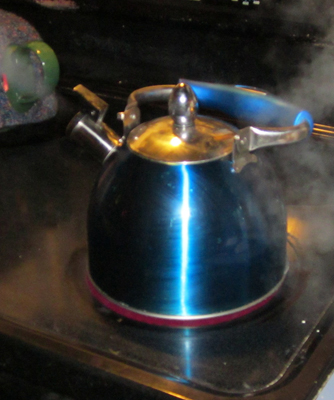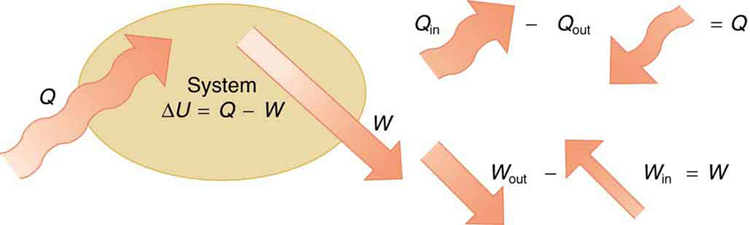# 15.1 The first law of thermodynamics

 Page 1 / 10

## Learning objectives

By the end of this section, you will be able to:

• Define the first law of thermodynamics.
• Describe how conservation of energy relates to the first law of thermodynamics.
• Identify instances of the first law of thermodynamics working in everyday situations, including biological metabolism.
• Calculate changes in the internal energy of a system, after accounting for heat transfer and work done.

The information presented in this section supports the following AP® learning objectives and science practices:

• 4.C.3.1 The student is able to make predictions about the direction of energy transfer due to temperature differences based on interactions at the microscopic level. (S.P. 6.1)
• 5.B.4.1 The student is able to describe and make predictions about the internal energy of systems. (S.P. 6.4, 7.2)
• 5.B.7.1 The student is able to predict qualitative changes in the internal energy of a thermodynamic system involving transfer of energy due to heat or work done and justify those predictions in terms of conservation of energy principles. (S.P. 6.4, 7.2)This boiling tea kettle represents energy in motion. The water in the kettle is turning to water vapor because heat is being transferred from the stove to the kettle. As the entire system gets hotter, work is done—from the evaporation of the water to the whistling of the kettle. (credit: Gina Hamilton)

If we are interested in how heat transfer is converted into doing work, then the conservation of energy principle is important. The first law of thermodynamics applies the conservation of energy principle to systems where heat transfer and doing work are the methods of transferring energy into and out of the system. The first law of thermodynamics    states that the change in internal energy of a system equals the net heat transfer into the system minus the net work done by the system. In equation form, the first law of thermodynamics is

$\Delta U=Q-W\text{.}$

Here $\Delta U$ is the change in internal energy $U$ of the system. $Q$ is the net heat transferred into the system —that is, $Q$ is the sum of all heat transfer into and out of the system. $W$ is the net work done by the system —that is, $W$ is the sum of all work done on or by the system. We use the following sign conventions: if $Q$ is positive, then there is a net heat transfer into the system; if $W$ is positive, then there is net work done by the system. So positive $Q$ adds energy to the system and positive $W$ takes energy from the system. Thus $\Delta U=Q-W$ . Note also that if more heat transfer into the system occurs than work done, the difference is stored as internal energy. Heat engines are a good example of this—heat transfer into them takes place so that they can do work. (See [link] .) We will now examine $Q$ , $W$ , and $\Delta U$ further.The first law of thermodynamics is the conservation-of-energy principle stated for a system where heat and work are the methods of transferring energy for a system in thermal equilibrium. Q size 12{Q} {} represents the net heat transfer—it is the sum of all heat transfers into and out of the system. Q size 12{Q} {} is positive for net heat transfer into the system. W size 12{W} {} is the total work done on and by the system. W size 12{W} {} is positive when more work is done by the system than on it. The change in the internal energy of the system, Δ U size 12{ΔU} {} , is related to heat and work by the first law of thermodynamics, Δ U = Q − W size 12{ΔU=Q - W} {} .

Why does earth exert only a tiny downward pull?
hello
Islam
Why is light bright?
an 8.0 capacitor is connected by to the terminals of 60Hz whoes rms voltage is 150v. a.find the capacity reactance and rms to the circuit
thanks so much. i undersooth well
what is physics
is the study of matter in relation to energy
Kintu
a submersible pump is dropped a borehole and hits the level of water at the bottom of the borehole 5 seconds later.determine the level of water in the borehole
what is power?
power P = Work done per second W/ t. It means the more power, the stronger machine
Sphere
e.g. heart Uses 2 W per beat.
Rohit
A spherica, concave shaving mirror has a radius of curvature of 32 cm .what is the magnification of a persons face. when it is 12cm to the left of the vertex of the mirror
did you solve?
Shii
1.75cm
Ridwan
my name is Abu m.konnek I am a student of a electrical engineer and I want you to help me
Abu
the magnification k = f/(f-d) with focus f = R/2 =16 cm; d =12 cm k = 16/4 =4
Sphere
what do we call velocity
Kings
A weather vane is some sort of directional arrow parallel to the ground that may rotate freely in a horizontal plane. A typical weather vane has a large cross-sectional area perpendicular to the direction the arrow is pointing, like a “One Way” street sign. The purpose of the weather vane is to indicate the direction of the wind. As wind blows pa
hi
Godfred
Godfred
If a prism is fully imersed in water then the ray of light will normally dispersed or their is any difference?
the same behavior thru the prism out or in water bud abbot
Ju
If this will experimented with a hollow(vaccum) prism in water then what will be result ?
Anurag
What was the previous far point of a patient who had laser correction that reduced the power of her eye by 7.00 D, producing a normal distant vision power of 50.0 D for her?
What is the far point of a person whose eyes have a relaxed power of 50.5 D?
Jaydie
What is the far point of a person whose eyes have a relaxed power of 50.5 D?
Jaydie
A young woman with normal distant vision has a 10.0% ability to accommodate (that is, increase) the power of her eyes. What is the closest object she can see clearly?
Jaydie
29/20 ? maybes
Ju
In what ways does physics affect the society both positively or negatively
how can I read physics...am finding it difficult to understand...pls help
try to read several books on phy don't just rely one. some authors explain better than other.
Ju
And don't forget to check out YouTube videos on the subject. Videos offer a different visual way to learn easier.
Ju
hope that helps
Ju
I have a exam on 12 february
what is velocity
Jiti
the speed of something in a given direction.
JuBy